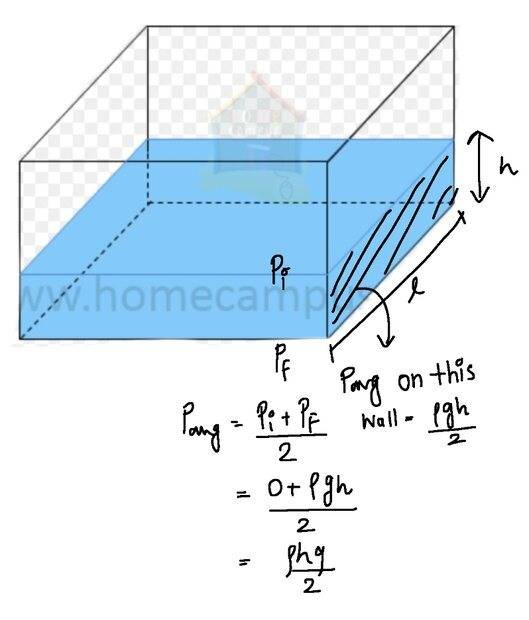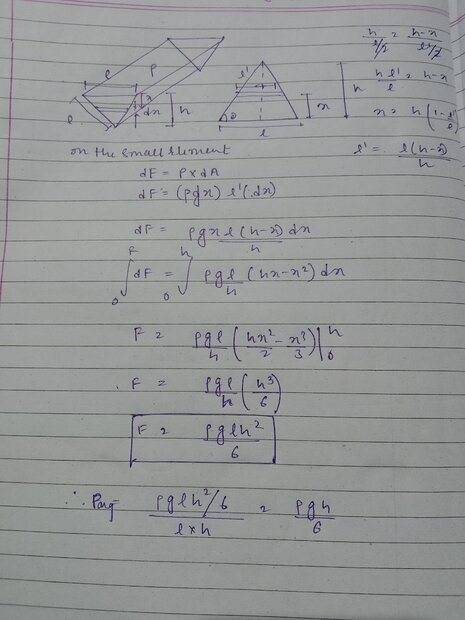# Pressure due to a Liquid on container Wall

SpectraPhy09
Homework Statement:
What is the average pressure on the triangular wall by the liquid ?
given :
density of liquid p
gravitational acc is g
For Dimensions of container please check the figure(i have attached please Check)
.( Assume Patm = 0)
Relevant Equations:
Pressure at a point, x distance below the free surface of the liquid = pgx
I have just started this topic so i Don't have much clearity about it .
In our school it was tought that
Pavg = (Pi + Pf)/2 ...(i)
If we have a cuboidal shape container then it should be pgh/2 ryt ?But also Pavg = Ftotal on the wall /A ...(ii)
= (pgh²l/2)/lh = pgh/2

It it was a triangular wall then Pavg comes different

Using (i) Pavg = pgh/2
(ii) Pavg = pgh/3

Which is correct ? 😓

Using

#### Attachments

Homework Helper
Gold Member
2022 Award
Ftotal on the wall /A ...(ii)
= (pgh²l/2)/lh
How do you get that for the total force?
And are you saying lh is the area?

SpectraPhy09
@haruspex
Total force on a container wall
= Pressure at geometrical centre × Area of the Surface Or Pavg × Area
For Cuboidal Container
Geometric centre is at h/2 from surface
P = pgh/2
Area = l × h
F = pgh²l/2
For Triangular Surface shaped Container
Geometric centre is at centroid i.e h/3 from surface
P = pgh/3
A = h×l/2

Homework Helper
Gold Member
2022 Award
Geometric centre is at h/2 from surface
Not so.

Mentor
The average pressure on a flat surface is the total force on the surface divided by total area of the surface. What is the total area of the surface that you are interested in? Do you know how to determine the total pressure force on this surface?

SpectraPhy09
The average pressure on a flat surface is the total force on the surface divided by total area of the surface. What is the total area of the surface that you are interested in? Do you know how to determine the total pressure force on this surface?
Yes got it now
I was finding Pavg on the triangular wall

What I could do is this -There's a correction in the image
Pavg = (pglh^2/6)/(lh/2) = pgh/3
now is it correct ?

Not so.
But why? For a rectangular surface isn't the Geomecal center at h/2?